# Chapter 2 Polynomials Important Questions for CBSE Class 10 Maths Board Exams

This page contains Important Questions for Class 10 Maths Chapter 2 Polynomials, which will help the students to prepare for the CBSE Class 10 maths Board exam 2022-23. It help the doing better in their maths paper. Extra questions of Chapter 2 Polynomials given here which are based on the pattern of CBSE NCERT book. Students will learn about the entire syllabus and learn how to solve problems in preparation for the exams.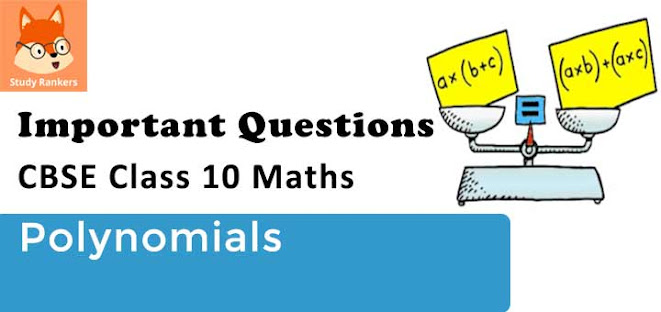## Important Questions for Chapter 2 Polynomials Class 10 Maths

Important Questions Class 10 Maths contains all types of questions which could be asked in the examination. We have included short answer questions as well as long answer questions for Chapter 2 Polynomials. For more exercise, students can also refer to the Polynomials NCERT Solutions. Students can solve these questions and check their answers on the website. All the questions are solved in detail.

### Polynomials Class 10 Maths Important Questions Very Short Answer (1 Mark)

1. If α and β are the roots of ax2 - bx + c = 0 (a≠ 0), then calculate α+β.

Solution

We know that,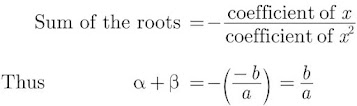2. If -1 is a zero of the polynomial f(x) = x2 - 7x - 8, then calculate the other zero.

Solution

We have f(x) = x2 - 7x - 8

Let other zero be k, then we have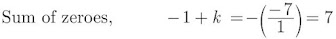⇒ k = 8

3. If the sum of zeroes of the quadratic polynomial 3x2 – kx + 6 is 3, then find the value of k.

Solution

Here a = 3, b = -k, c = 6

Sum of the zeroes, (α + β) = ba = 3 (given)

(k)3 = 3

⇒ k = 9

4. A quadratic polynomial, whose zeroes are -4 and -5, is ….

Solution

x2 + 9x + 20 is the required polynomial.

5. Polynomials of degrees 1, 2 and 3 are called ........, ....... and ........ polynomials respectively.

Solution

6. The algebraic expression in which the variable has non-negative integral exponents only is called ..........

Solution

Polynomial

7. If α and β are the zeroes of a polynomial such that α + β = -6 and αβ = 5, then find the polynomial.

Solution

Quadratic polynomial is x2 – Sx + P = 0

⇒ x2 – (-6)x + 5 = 0

⇒ x2 + 6x + 5 = 0

### Polynomials Class 10 Maths Important Questions Short Answer-I (2 Marks)

8. Find all the zeroes of f(x) = x2 - 2x

Solution

f(x) = x2 - 2x
= x(x-2)
Substituting f(x) = 0, and solving we get, x= 0, 2x
Hence, zeroes are 0 and 2.

9. Find the condition that zeroes of polynomial p(x) = ax2 + bx + c are reciprocal of each other.

Solution

Let α and 1/α be the zeroes of f(x).
f(a) = ax2 + bx + c …(given)
Product of zeroes = ca
⇒ α × 1/α = ca
⇒ 1 = ca
⇒ a = c (Required condition)
Coefficient of x2 = Constant term

10. Find the zeroes of the quadratic polynomial √3x2 - 8x + 4√3

Solution

p(x) = √3 x2 - 8x + 4√3

= √3 x2 - 6x – 2x + 4√3

= √3x(x - 2√3) - 2(x - 2√3)

(√3 x -2) (x - 2√3)

Substituting p(x) = 0, we have

(√3 x -2) (x - 2√3)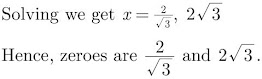11. Form a quadratic polynomial p(x) with 3 and -2/5 as sum and product of its zeroes, respectively.

Solution

Sum of zeroes, α+β = 3

Product of zeroes αβ = -2/5

Now,

p(x) = x2 - (α+β)x + αβ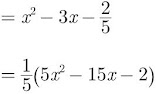12. Find a quadratic polynomial, the sum and product of whose zeroes are 0 and -√2 respectively.

Solution

x2 – (Sum of zeroes) x + (Product of zeroes)
= x2 – (0)x + (-√2)
= x2 – √2

13. If the zeroes of the polynomial x2 + px + q are double in value to the zeroes of 2x2 – 5x – 3, find the value of p and q.

Solution

We have, 2x2 – 5x – 3 = 0
= 2x2 – 6x + x – 3
= 2x(x – 3) + 1(x – 3)
= (x – 3) (2x + 1)
Zeroes are:
x – 3 = 0 or 2x + 1 = 0
⇒ x = 3 or x = −12
Since, the zeroes of required polynomial is double of given polynomial.
Zeroes of the required polynomial are:
3×2, (−12×2) i.e. 6, -1
Sum of zeroes = 6 + (-1) = 5
Product of zeroes = 6 × (-1) = -6
Quadratic polynomial is x2 – Sx + P
⇒ x2 – 5x – 6 …(i)
Comparing (i) with x2 + px + q
p = -5, q = -6

14. If m and n are the zeroes of the polynomial 3x2 + 11x - 4, find the value of m/n + n/m.

Solution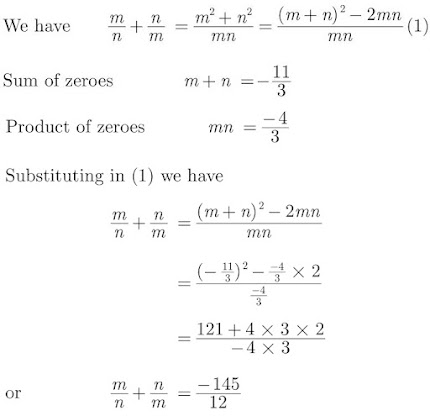15. If p and q are the zeroes of polynomial f(x)  = 2x2 - 7x + 3, find the value of p2 + q2.
Solution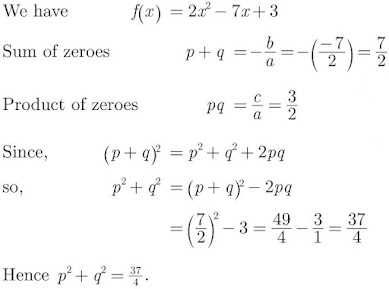16. Find the zeroes of p(x) = 2x2 – x – 6 and verify the relationship of zeroes with these co-efficients.

Solution

p(x) = 2x2 – x – 6 [Given]
= 2x2 – 4x + 3x – 6
= 2x (x – 2) + 3 (x – 2)
= (x – 2) (2x + 3)
Zeroes are:
x – 2 = 0 or 2x + 3 = 0
x = 2 or x = −32
Verification:
Here a = 2, b = -1, c = -6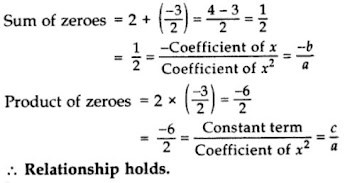17. If one zero of the polynomial 2x2 + 3x + λ is 1/2, find the value of λ and the other zero.

Solution

Let, the zero of 2x2 + 3x + λ be 1/2 and β.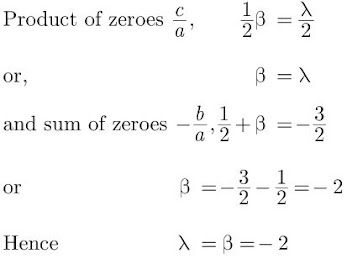Thus, the other zero is -2.

18. If α and β are zeroes of x2 - (k-6)x + 2(2k-1), find the value of k if α+β = 1/2 αβ.
Solution
We have,
p(x) = x2 - (k-6)x + 2(2k-1)
Since,
α, β are the zeroes of polynomial p(x), we get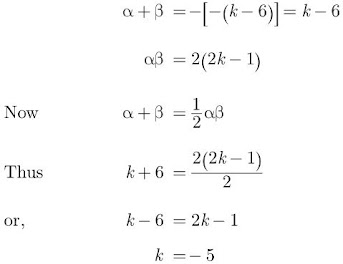Hence, the value of k is -5.

19. What must be subtracted from the polynomial f(x) = x4 + 2x3 – 13x2 – 12x + 21 so that the resulting polynomial is exactly divisible by x2 – 4x + 3?

Solution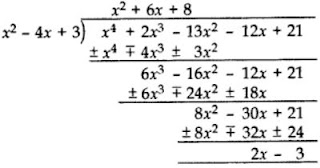(2x – 3) should be subtracted from x4 + 2x3 – 13x2 – 12x + 21.

### Polynomials Class 10 Maths Important Questions Short Answer-II (3 Marks)

20. Find a quadratic polynomial whose zeroes are reciprocals of the zeroes of the polynomial f(x) = ax2 + bx + c, a≠0, c≠0.

Solution

Let α and β be zeros of the given polynomial ax2 + bx + c.

α+β = -b/a and αβ = c/a

Let 1/α and 1/β be the zeros of new polynomial then we have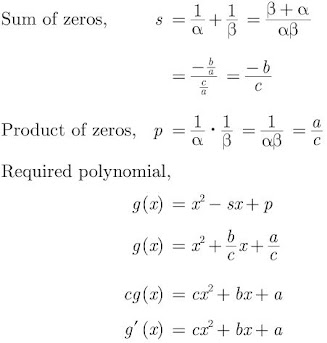21. Verify whether 2, 3 and 1/2 are the zeroes of the polynomial p(x) = 2x3 - 11x2 + 17x - 6.
Solution
If 2, 3 and 1/2 are the zeroes of the polynomial p(x), then these must satisfy p(x) = 0.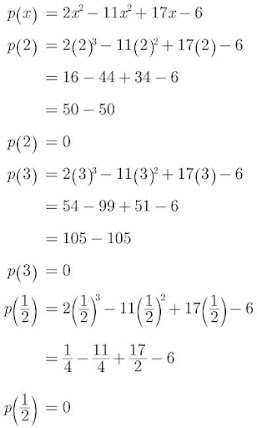Hence, 2, 3, and 1/2 are the zeroes of p(x).

22. Show that 12 and −32 are the zeroes of the polynomial 4x2 + 4x – 3 and verify the relationship between zeroes and co-efficients of polynomial.
Solution
Let P(x) = 4x2 + 4x – 3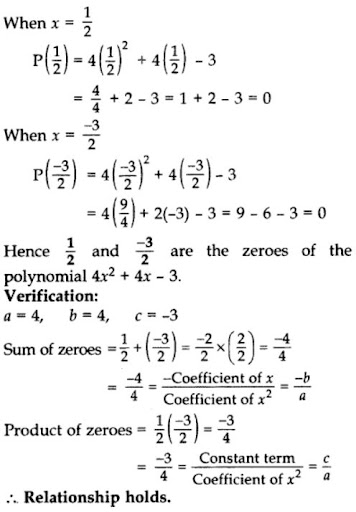23. Find a quadratic polynomial, the sum and product of whose zeroes are -8 and 12 respectively. Hence find the zeroes.

Solution

Let Sum of zeroes (α + β) = S = -8  [Given]
Product of zeroes (αβ) = P = 12  [Given]
Quadratic polynomial is x2 – Sx + P
= x2 – (-8)x + 12
= x2 + 8x + 12
= x2 + 6x + 2x + 12
= x(x + 6) + 2(x + 6)
= (x + 2)(x + 6)
Zeroes are:
x + 2 = 0 or x + 6 = 0
x = -2 or x = -6

24. Find the zeroes of the quadratic polynomial 5x2 + 8x - 4 and verify the relationship between the zeroes and the coefficients of the polynomial.

Solution

We have,

p(x) = 5x2 + 8x - 4 = 0

= 5x2 + 10x -2x - 4 = 0

= 5x(x+2) -2(x+2) = 0

= (x+2) (5x-2)

Substituting p(x) = 0 we get zeroes as 2 - and 2/5.

Verification :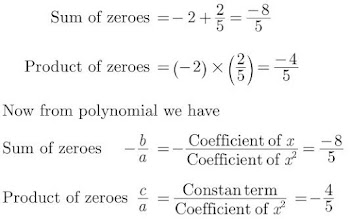25. Check whether polynomial x – 1 is a factor of the polynomial x3 – 8x2 + 19x – 12. Verify by division algorithm.

Solution

Let P(x) = x3 – 8x2 + 19x – 12
Put x = 1
P(1) = (1)3 – 8(1)2 + 19(1) – 12
= 1 – 8 + 19 – 12
= 20 – 20
= 0
Remainder = 0
(x – 1) is a factor of P(x).

Verification: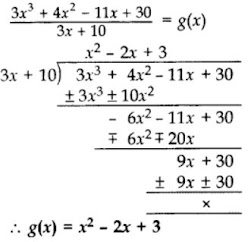Since remainder = 0
(x – 1) is a factor of P(x).

26. When p(x) = x2 + 7x + 9 is divisible by g(x), we get (x+2) and -1 as the quotient and remainder respectively, find g(x).

Solution

We have,

p(x) = x2 + 7x + 9

q(x) = x+2

r(x) = -1

Now,

p(x) = g(x) q(x) + r(x)

x2 + 7x + 9 = g(x) (x+2) -1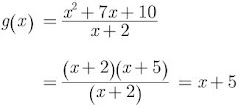Thus, g(x) = x+5

27. Find the value for k for which x4 + 10x3 + 25x2 + 15x + k is exactly divisible by x+7.

Solution

We have,

f(x) = x4 + 10x3 + 25x2 + 15x + k

If x+7 is a factor then -7 is a zero of f(x) and x = -7 satisfy f(x) = 0.

Thus substituting x = -7 in f(x) and equating to zero we have,

(-7)4 + 10(-7)3 + 25(-7)2 + 15(-7) + k = 0

⇒ 2401 - 3430 + 1225 - 105 + k = 0

⇒ 3626 - 3535 + k = 0

⇒ 91 + k = 0

⇒ k = -91

28. If the squared difference of the zeroes of the quadratic polynomial f(x) = x2 + px + 45 is equal to 144, find the value of p.

Solution

We have,

f(x) = x2 + px + 45

Let α and β be the zeroes of the given quadratic polynomial.

Sum of zeroes, α+β = −p

Product of zeroes, αβ = 45

Given,

(α-β)2 = 144

⇒ (α+β)2 + 4αβ = 144

Substituting value of α+β and αβ we get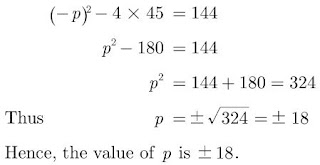### Polynomials Class 10 Maths Important Questions Long Answer (4 Marks)

29. Divide 4x3 + 2x2 + 5x – 6 by 2x2 + 1 + 3x and verify the division algorithm. (2013)

Solution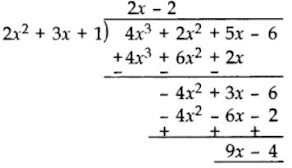Quotient = 2x – 2
Remainder = 9x – 4
Verification:
Divisor × Quotient + Remainder
= (2x2 + 3x + 1) × (2x – 2) + 9x – 4
= 4x3 – 4x2 + 6x2 – 6x + 2x – 2 + 9x – 4
= 4x3 + 2x2 + 5x – 6
= Dividend

30. If α and β are the zeroes of the polynomial p(x) = 2x2 + 5x + k satisfying the relation, α2 + β2 + αβ = 21/4, then find the value of k.

Solution

We have,

p(x) = 2x2 + 5x + k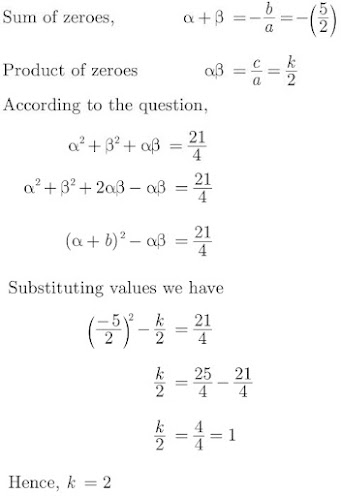31. If α and β are the zeroes of polynomial p(x) = 3x2 + 2x + 1, find the polynomial whose zeroes are 1-α/1+α and 1-β/1+β.
Solution
We have,
p(x) = 3x2 + 2x + 1
Since α and β are the zeroes of polynomial 3x2 + 2x + 1, we have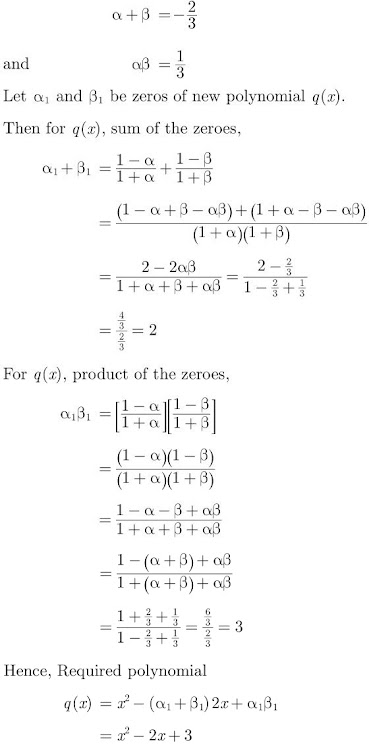32. If α and β are the zeroes of the polynomial x2 + 4x + 3, find the polynomial whose zeroes are 1 + β/α and 1 + α/β.
Solution
We have,
p(x) = x2 + 4x + 3
Since α and β are the zeroes of the quadratic polynomial x2 + 4x + 3,
So,
α + β = −4
and αβ = 3
Let α1 and β1 be zeros of new polynomial q(x).
Then for q(x), sum of the zeroes,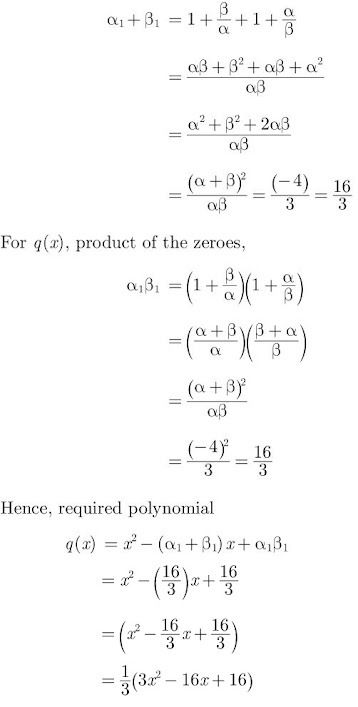33. If α and β are zeroes of p(x) = kx2 + 4x + 4, such that α2 + β2 = 24, find k.

Solution

We have, p(x) = kx2 + 4x + 4
Here a = k, b = 4, c = 4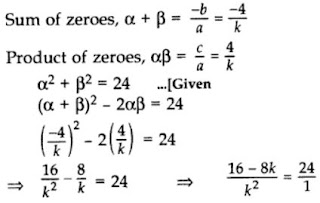⇒ 24k2 = 16 – 8k
⇒ 24k2 + 8k – 16 = 0
⇒ 3k2 + k – 2 = 0 [Dividing both sides by 8]
⇒ 3k2 + 3k – 2k – 2 = 0
⇒ 3k(k + 1) – 2(k + 1) = 0
⇒ (k + 1)(3k – 2) = 0
⇒ k + 1 = 0 or 3k – 2 = 0
⇒ k = -1 or k = 23

34. If β and 1/β are zeroes of the polynomial (a2 + a)x2 + 61x + 6a. Find the value of β and α.
Solution
We have,
p(x) = (a2 + a)x2 + 61x + 6a
Since β and 1/β are the zeroes of polynomial, p(x)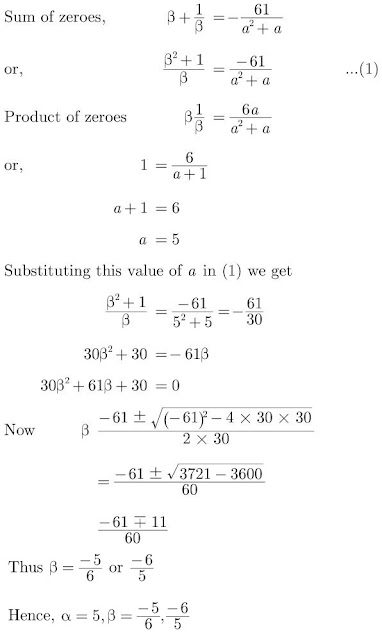35. If the polynomial (x4 + 2x3 + 8x2 + 12x + 18) is divided by another polynomial (x2 + 5), the remainder comes out to be (px + q), find the values of p and q.

Solution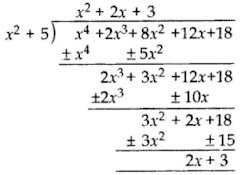Remainder = 2x + 3
px + q = 2x + 3
p = 2 and q = 3

36. If α and β are the zeroes the polynomial 2x2 - 4x + 5, find the values of

(i) α22
(ii) 1/α + 1/β
(iii) (α-β)2
(iv) 1/α2 + 1/β2
(v) α2 + β2

Solution

We have,
p(x) = 2x2 - 4x + 5p
If α and β are then zeroes of p(x) = 2x2 - 4x + 5, then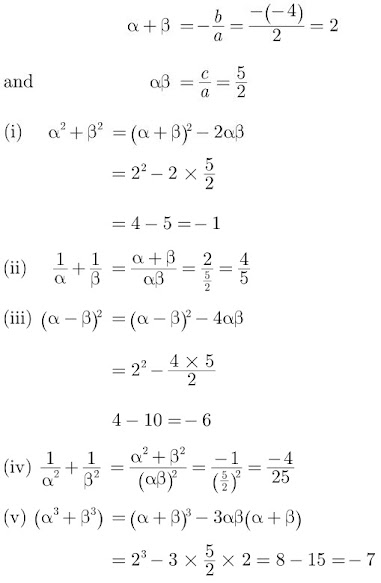37. If p(x) = x3 – 2x2 + kx + 5 is divided by (x – 2), the remainder is 11. Find k. Hence find all the zeroes of x3 + kx2 + 3x + 1.

Solution

p(x) = x3 – 2x2 + kx + 5,
When x – 2,
p(2) = (2)3 – 2(2)2 + k(2) + 5
⇒ 11 = 8 – 8 + 2k + 5
⇒ 11 – 5 = 2k
⇒ 6 = 2k
⇒ k = 3
Let q(x) = x3 + kx2 + 3x + 1
= x3 + 3x2 + 3x + 1
= x3 + 1 + 3x2 + 3x
= (x)3 + (1)3 + 3x(x + 1)
= (x + 1)3
= (x + 1) (x + 1) (x + 1) [∵ a3 + b3 + 3ab (a + b) = (a + b)3]
All zeroes are:
x + 1 = 0 ⇒ x = -1
x + 1 = 0 ⇒ x = -1
x + 1 = 0 ⇒ x = -1
Hence zeroes are -1, -1 and -1.